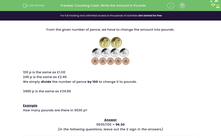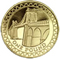# Convert Pence into Pounds

In this worksheet, students will convert an amount given in pence into pounds.Key stage:  KS 2

Curriculum topic:   Measurement

Curriculum subtopic:   Use Different Measures

Popular topics:   Money worksheets

Difficulty level:#### Worksheet Overview

From the given number of pence, we have to change the amount into pounds and pence.100p is the same as £1.00

246p is the same as £2.46

We simply divide the number of pence by 100 to change it to pounds because there are 100 pence in a pound.

3486p is the same as £34.86

Example

How many pounds and pence are there in 9630p?

9630 ÷ 100 = 96.30

An important point to notice is that the decimal point separates the pounds and the pence!!